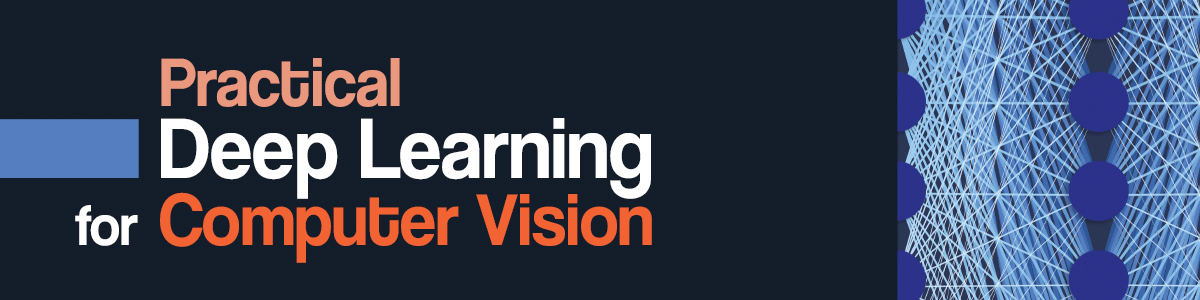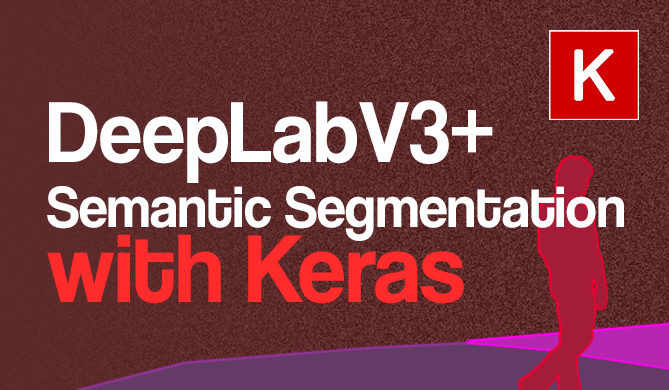What is 'from_logits=True' in Keras/TensorFlow Loss Functions?

# What is 'from_logits=True' in Keras/TensorFlow Loss Functions?Deep Learning frameworks like Keras lower the barrier to entry for the masses and democratize the development of DL models to unexperienced folk, who can rely on reasonable defaults and simplified APIs to bear the brunt of heavy lifting, and produce decent results.

A common confusion arises between newer deep learning practitioners when using Keras loss functions for classification, such as CategoricalCrossentropy and SparseCategoricalCrossentropy:

loss = keras.losses.SparseCategoricalCrossentropy(from_logits=True)
# Or
loss = keras.losses.SparseCategoricalCrossentropy(from_logits=False)


What does the from_logits flag refer to?

The answer is fairly simple, but requires a look at the output of the network we're trying to grade using the loss function.

## Logits and SoftMax Probabilities

Long story short:

Probabilities are normalized - i.e. have a range between [0..1]. Logits aren't normalized, and can have a range between [-inf...+inf].

Depending on the output layer of your network:

output = keras.layers.Dense(n, activation='softmax')(x)
# Or
output = keras.layers.Dense(n)(x)


The output of the Dense layer will either return:

• probabilities: The output is passed through a SoftMax function which normalizes the output into a set of probabilities over n, that all add up to 1.
• logits: n activations.

This misconception possibly arises from the short-hand syntax that allows you to add an activation to a layer, seemingly as a single layer, even though it's just shorthand for:

output = keras.layers.Dense(n, activation='softmax')(x)
# Equivalent to
dense = keras.layers.Dense(n)(x)
output = keras.layers.Activation('softmax')(dense)


Your loss function has to be informed as to whether it should expect a normalized distribution (output passed through a SoftMax function) or logits. Hence, the from_logits flag!

## When Should from_logits=True?

If your output layer has a 'softmax' activation, from_logits should be False. If your output layer doesn't have a 'softmax' activation, from_logits should be True.

If your network normalizes the output probabilities, your loss function should set from_logits to False, as it's not accepting logits. This is also the default value of all loss classes that accept the flag, as most people add an activation='softmax' to their output layers:

model = keras.Sequential([
keras.layers.Input(shape=(10, 1)),
# Outputs normalized probability - from_logits=False
keras.layers.Dense(10, activation='softmax')
])

input_data = tf.random.uniform(shape=[1, 1])
output = model(input_data)
print(output)


This results in:

tf.Tensor(
[[[0.12467965 0.10423233 0.10054766 0.09162105 0.09144577 0.07093797
0.12523937 0.11292477 0.06583504 0.11253635]]], shape=(1, 1, 10), dtype=float32)


Since this network results in a normalized distribution - when comparing the outputs with target outputs, and grading them via a classification loss function (for the appropriate task) - you should set from_logits to False, or let the default value stay.

On the other hand, if your network doesn't apply SoftMax on the output:

model = keras.Sequential([
keras.layers.Input(shape=(10, 1)),
# Outputs logits - from_logits=True
keras.layers.Dense(10)
])

input_data = tf.random.uniform(shape=[1, 1])
output = model(input_data)
print(output)


This results in:

tf.Tensor(
[[[-0.06081138  0.04154852  0.00153442  0.0705068  -0.01139916
0.08506121  0.1211026  -0.10112958 -0.03410497  0.08653068]]], shape=(1, 1, 10), dtype=float32)


You'd need to set from_logits to True for the loss function to properly treat the outputs.

## When to Use SoftMax on the Output?

Most practitioners apply SoftMax on the output to give a normalized probability distribution, as this is in many cases what you'll use a network for - especially in simplified educational material. However, in some cases, you don't want to apply the function to the output, to process it in a different way before applying either SoftMax or another function.

A notable example comes from NLP models, in which a really the probability over a large vocabulary can be present in the output tensor. Applying SoftMax over all of them and greedily getting the argmax typically doesn't produce very good results.

Free eBook: Git Essentials

Check out our hands-on, practical guide to learning Git, with best-practices, industry-accepted standards, and included cheat sheet. Stop Googling Git commands and actually learn it!

However, if you observe the logits, extract the Top-K (where K can be any number but is typically somewhere between [0...10]), and only then applying SoftMax to the top-k possible tokens in the vocabulary shifts the distribution significantly, and usually produces more realistic results.

This is known as Top-K sampling, and while it isn't the ideal strategy, usually significantly outperforms greedy sampling.Advice: To see this principle in action in an end-to-end project that teaches you the crux of building autoregressive language models with TensorFlow and Keras - read our "5-Line GPT-Style Text Generation in Python with TensorFlow/Keras"!

## Going Further - Practical Deep Learning for Computer Vision

Your inquisitive nature makes you want to go further? We recommend checking out our Course: "Practical Deep Learning for Computer Vision with Python".### Another Computer Vision Course?

We won't be doing classification of MNIST digits or MNIST fashion. They served their part a long time ago. Too many learning resources are focusing on basic datasets and basic architectures before letting advanced black-box architectures shoulder the burden of performance.

We want to focus on demystification, practicality, understanding, intuition and real projects. Want to learn how you can make a difference? We'll take you on a ride from the way our brains process images to writing a research-grade deep learning classifier for breast cancer to deep learning networks that "hallucinate", teaching you the principles and theory through practical work, equipping you with the know-how and tools to become an expert at applying deep learning to solve computer vision.

### What's inside?

• The first principles of vision and how computers can be taught to "see"
• Different tasks and applications of computer vision
• The tools of the trade that will make your work easier
• Finding, creating and utilizing datasets for computer vision
• The theory and application of Convolutional Neural Networks
• Handling domain shift, co-occurrence, and other biases in datasets
• Transfer Learning and utilizing others' training time and computational resources for your benefit
• Building and training a state-of-the-art breast cancer classifier
• How to apply a healthy dose of skepticism to mainstream ideas and understand the implications of widely adopted techniques
• Visualizing a ConvNet's "concept space" using t-SNE and PCA
• Case studies of how companies use computer vision techniques to achieve better results
• Proper model evaluation, latent space visualization and identifying the model's attention
• Performing domain research, processing your own datasets and establishing model tests
• Cutting-edge architectures, the progression of ideas, what makes them unique and how to implement them
• KerasCV - a WIP library for creating state of the art pipelines and models
• How to parse and read papers and implement them yourself
• Selecting models depending on your application
• Creating an end-to-end machine learning pipeline
• Landscape and intuition on object detection with Faster R-CNNs, RetinaNets, SSDs and YOLO
• Instance and semantic segmentation
• Real-Time Object Recognition with YOLOv5
• Training YOLOv5 Object Detectors
• Working with Transformers using KerasNLP (industry-strength WIP library)
• Integrating Transformers with ConvNets to generate captions of images
• DeepDream

## Conclusion

In this short guide, we've taken a look at the from_logits argument for Keras loss classes, which oftentimes raise questions with newer practitioners.

The confusion possibly arises from the short-hand syntax that allows the addition of activation layers on top of other layers, within the definition of a layer itself. We've finally taken a look at when the argument should be set to True or False, and when an output should be left as logits or passed through an activation function such as SoftMax.

Last Updated: August 30th, 2022

Get tutorials, guides, and dev jobs in your inbox.

David LandupAuthor

Entrepreneur, Software and Machine Learning Engineer, with a deep fascination towards the application of Computation and Deep Learning in Life Sciences (Bioinformatics, Drug Discovery, Genomics), Neuroscience (Computational Neuroscience), robotics and BCIs.

Great passion for accessible education and promotion of reason, science, humanism, and progress.Project

### DeepLabV3+ Semantic Segmentation with Keras

# tensorflow# keras# python# machine learning

Semantic segmentation is the process of segmenting an image into classes - effectively, performing pixel-level classification. Color edges don't necessarily have to be the boundaries...David Landup
DetailsProject

### Building Your First Convolutional Neural Network With Keras

# artificial intelligence# machine learning# keras# deep learning

Most resources start with pristine datasets, start at importing and finish at validation. There's much more to know. Why was a class predicted? Where was...David Landup
Details